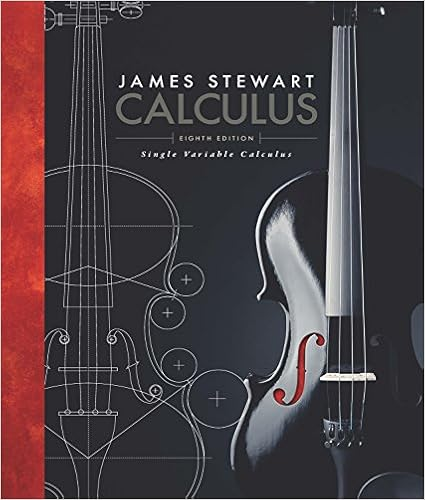# How does the area of the rectangle relate to the

• Assignment
• 7
• 92% (25) 23 out of 25 people found this document helpful

This preview shows page 4 - 7 out of 7 pages.

##### We have textbook solutions for you!
The document you are viewing contains questions related to this textbook.The document you are viewing contains questions related to this textbook.
Chapter 10 / Exercise 34
Single Variable Calculus
StewartExpert Verified
B. How does the area of the rectangle relate to the lengths of each side? ___________ 2.Observe: A block is an example of a rectangular prism. A rectangular prism has six rectangular faces. Look at the block shown below. Each cube inside the block has a 1-cm side and a volume of 1 cm3, or 1 mL. A.What are the length, width, and height of the block?Length: _____ Width: _____ Height: _____B.Multiply these three dimensions. What is the product of thelength, width, and height? __________C. How many cubic centimeters are in the block? __________ 3.Measure: Just as the area of a rectangle is the product of its length and width, the volume of a rectangular prism is equal to the product of its length, width, and height. In the Gizmo, place the ruler over the blockA.What are the length, width, and height of the block?. Length: _____ Width: _____ Height: _____B.What is the volume of the block? __________ (Activity B continued on next page)
##### We have textbook solutions for you!
The document you are viewing contains questions related to this textbook.The document you are viewing contains questions related to this textbook.
Chapter 10 / Exercise 34
Single Variable Calculus
StewartExpert Verified
Activity B (continued from previous page)4.Measure: Return the block to the cabinet and drag out the large sphere. The volume of a sphere is calculated using the following formula: VSphere = 4πr3/ The symbol πrepresents the number pi, which is about 3.14. The letter r stands for the radius of the sphere, which is the distance from the center of a sphere to its surface. The radius is exactly half of the diameter, which is the distance across the sphere. (The diameter is also equal to the length, width, and height of the sphere.) A.Place the ruler over the sphere. What is the diameter of the sphere? __________ B.What is the radius of the sphere? __________ C.What is the volume of the sphere? __________ 5.Measure: Return the large sphere to the cabinet and drag out the small marble. Use the ruler and your calculator to find the volume of the marble. Show your work. Volume of the marble: __________ 6.Further practice: Select Practice. Do the next three problems, measuring the sphere, the marble, and the rectangular prism. (Note: The dimensions of each object vary slightly each time you go through the problems.) 3
_________________________________________________________________________ _________________________________________________________________________ _________________________________________________________________________ _________________________________________________________________________ Activity C: Water displacement Get the Gizmo ready: Select Free Exploration. Return all objects to the cabinet. Drag the large sphere, the overflow cup, the 250-mL beaker, the 50-mL graduated cylinder, and the magnifier to the counter. Introduction: Have you ever climbed into a tub and seen the water rise? The amount the water rises is related to your sizethe bigger you are, the more the water will rise. This method, called water displacement, can be used to measure volume.
•••